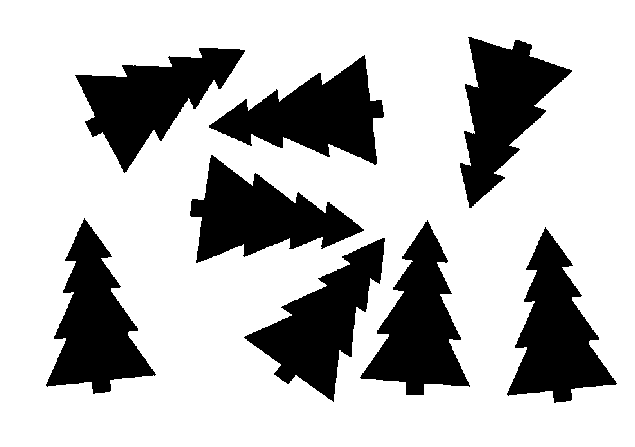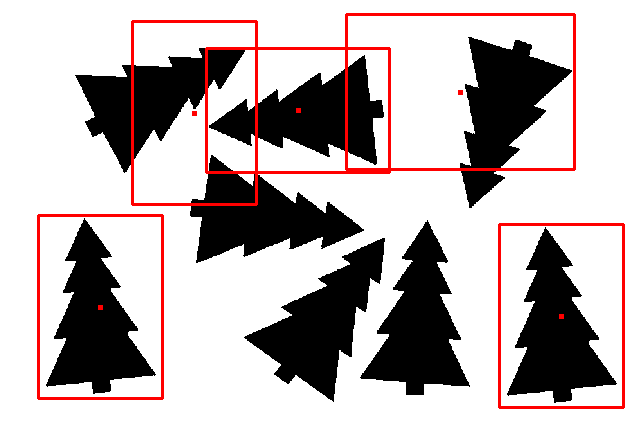# Generalized Hough Transform in OpenCV – set angle precision

I have a problem with Generalized Hough Transform (Guil version) in OpenCV. My code:

``````def generalized_Hough():
img_gray = cv2.cvtColor(img, cv2.COLOR_BGR2GRAY)

height, width = template.shape[:2]

edges = cv2.Canny(template, 200, 250)
ght = cv2.createGeneralizedHoughGuil()
ght.setTemplate(edges)

ght.setMinDist(100)
ght.setMinAngle(0)
ght.setMaxAngle(360)
ght.setAngleStep(1)
ght.setLevels(360)
ght.setMinScale(1)
ght.setMaxScale(1.3)
ght.setScaleStep(0.05)
ght.setAngleThresh(100)
ght.setScaleThresh(100)
ght.setPosThresh(100)
ght.setAngleEpsilon(1)
ght.setLevels(360)
ght.setXi(90)

positions = ght.detect(img_gray)

for position in positions:
center_col = int(position)
center_row = int(position)
scale = position
angle = int(position)

found_height = int(height * scale)
found_width = int(width * scale)

rectangle = ((center_col, center_row),
(found_width, found_height),
angle)

box = cv2.boxPoints(rectangle)
box = np.int0(box)
cv2.drawContours(img, [box], 0, (0, 0, 255), 2)

for i in range(-2, 3):
for j in range(-2, 3):
img[center_row + i, center_col + j] = 0, 0, 255

cv2.imwrite("results.png", img)
``````

So I read image and template, get template as Canny edges, then configure GHT (Guil version for scale and angle) and plot detections. The problem is that the results are always snapped to nearest 90 degrees, despite setting angle min, max and step to 0, 360 and 1, respectively:

``````[[294. 110.   1. 270.]
[100. 303.   1.   0.]
[561. 312.   1.   0.]
[461. 126.   1.  90.]
[194. 109.   1.   0.]]
``````

Image example:Template example:Results:It's clearly visible that upper left and upper right detections are in more or less correct places and scales, but angle is wrong. How can I fix that?